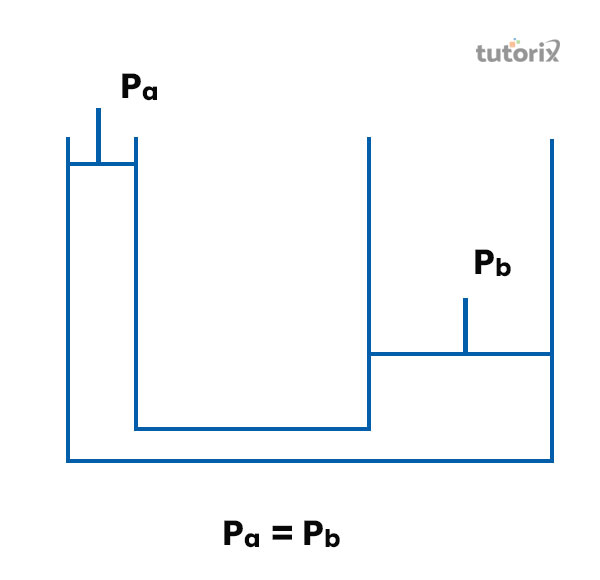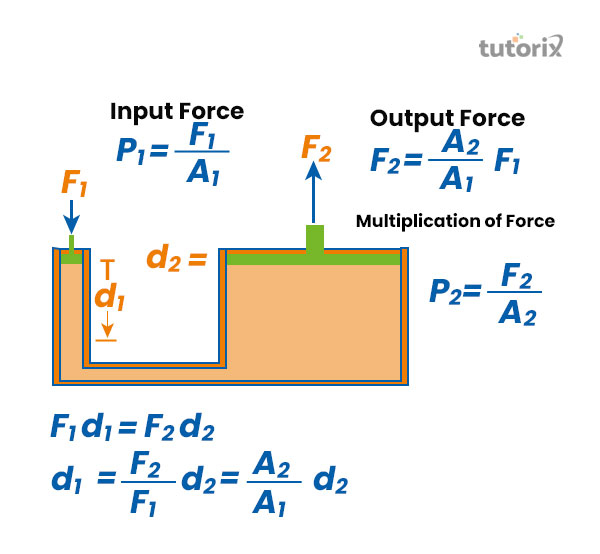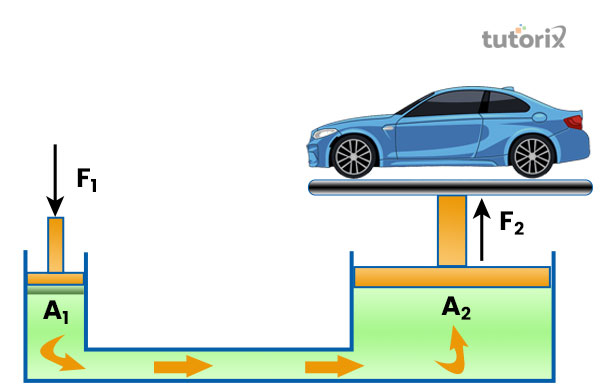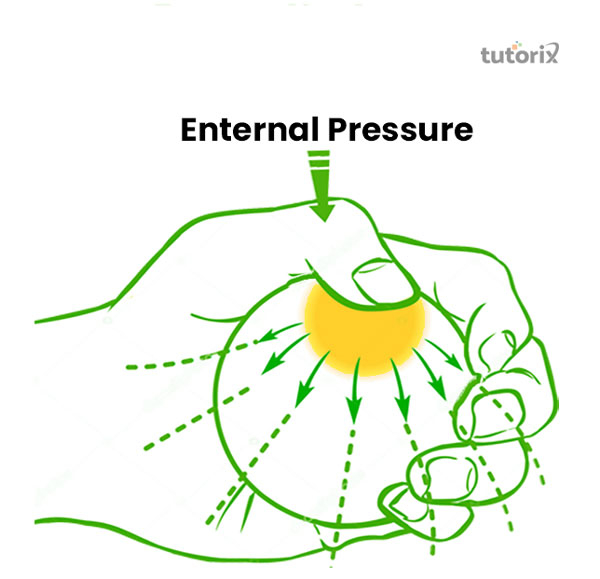# Pascals Law and Its Application

## Introduction

The principle of fluid pressure is transmitted in the machines defined in "Pascal’s law" and it discovers the force direction. The direction of the force in the fluid container gets directed to the pressure point and the change of fore get occurs everyplace. The principle of Pascal’s is associated with fluid mechanics. The application of this force transmission principle applies in modern days is the hydraulic system which is based on "Pascal’s law" published around 1650 by Blaise Pascal.

## Information Regarding Pascal's Law

"Pascal’s law" is regarded as a statement regarding the transformation of force where the point of pressure can travel without losing its force. In the real-world instance, if water is filled inside a closed container, the application of any point of force will be transferred to the container’s walls.

The pressure point in the fluid is sustainable and remains equal in all different directions. The process of transmitting the force makes energy construction (Hernandez, 2020). Moreover, the sum of the energies stays consistent. In the development of the pressure development, a resistance is constructed based on the output and input of the piston.Figure 1: A simpler form of "Pascal’s law"

The developed pressure will force it to move downside where the fluid acts equally in every part of the container. Fluid force cannot be constructed without resistance to flow. In this circumstance, the tool of piston’s outcome is connected with the resistance power. Whenever an external force is involved in any part of a container that is filled with liquid, it will be transferred undiminished in all the directions equally and the pressure will lie on the container walls.

Force (F1) applied from one side (input) will travel a distance (d1) that will be equal to another side (output) with the same amount of Force (F2).Figure 2: Explanation of "Pascal’s law"

## Applications of "Pascal’s law"

"Pascal’s law" is primarily utilised in the hydraulic systems in the modern world standard with the application of the force direction. The followings are the primary instances of "Pascal’s law":

### Hydraulic lift

Lift is utilised in the different functional processes for lifting heavy things in different scenarios. In different industries, transportations, constructional work, and others, the utilisation of lift is regular. According to the principles of Pascal, the pressure is determined by the objective inside the piston delivering enough amount of force to move an object upside or lift it by applying the pressure in the right direction. The hydraulic cylinder converts the fluid's power into strength and creates the pressure in the fluid, which is controlled by the hydraulic engine.

### Hydraulic jack

The process of a hydraulic jack works; it is followed to lift a portion of heavy objects to repair it. It is designed like a closed container which is contained with connected small and large cylinders. In the process of lifting something, once the handle is pushed, the valve closes which drives the one piston to exercise force on the large cylinder which additionally exerts strength to lift the object via constant up and down the motion by the handle (Farida et al. 2019). The smaller cylinder needs to travel a higher distance than the larger cylinder.Figure 3: Hydraulic Jack

### Hydraulics systems

In modern days, the hydraulic system works with the practical implication of the fluids in motion. It is associated with the theoretical foundation of "Pascal’s law". In different industries, Hydraulic systems are utilised for different purposes. In the practical world, some principles are even applied to gases which are usually varied in density. In different phases of industries, the use case of the hydraulic-power system has engaged more with the utilisation of technologies (Permana et al. 2021). In the automobile industry, the usefulness of the hydraulic system is applied in operating breaks and gears with the applied pressure formula.

### The way Hydraulic system works

The hydraulic lift permits techniques to use a small input power to construct a larger output power. This appears due to the force that is involved in one piston is equivalent to the force of the second piston. In other expressions, the force within the fluid stays undiminished and the pressure flowed upon the wall of the container.

### Example of Pascal’s Principle

Hydraulic techniques can improve or reduce the force involved in them. In order to make the strength more extensive, the pressure is involved in larger dimensions. For instance, if a 100-N power is involved in the left cylinder and the right cylinder has a location five times more strength, then the output strength is approximately 500 N. Hydraulic techniques are comparable to superficial levers, but they have the benefit that force can be sent via tortuously curved bars to several locations in one time.

### Proof of "Pascal’s law"

Considering a four cylindrical container is filled with fluid. The pistons of the container are A, B, C, and D, and the cross-sectional areas are respectively a, 2a, 3a, and 4a. All of the positions have the fitted in the right position where the piston (a), and is to be pushed with the applied force, (F). In this situation, the piston will be P = F/a. The other three pistons will move due to the applied pressure (F).

If the container is filled with liquid fully, then the pressure will be transmitted in all the different directions. In this situation, all the other pistons will move outside. In order to make them in their actual place, the force will be exerted on all of them. Hence, applying forces on a point will be directed to the occurrence of the container or object’s body in an equal way.Figure: 4: Pascal’s Principle of Force

## Conclusion

The classification of Pascal’s principle is used in a different use case where the application of force is important. The law to distribute the applied force equally uses the separation of energy. Moreover, according to the law, if A is the small cross-section of an objective, then the pressure P =F/A is transferred via the liquid to the more extensive cylinder attached with a larger piston of location B, which consequence as an application of the upward pressure of P × B. Consequently, the piston will be capable of sustaining a large force to lift something.

## FAQs

Q1. What is Pascal’s principle?

An external pressure applied on a container filled with liquid is transmitted in all directions on the walls of the container.

Q2. Can "Pascal’s law" is applied to gasses?

The primary application of "Pascal’s law" is on incompressible liquid; however, it can be applied to gasses.

Q3. What is the modern world application of "Pascal’s law"?

The hydraulic system is the best-applied technique in the modern world of "Pascal’s law".

Q4. What is the principle of “Pascal’s law”?

According to the statement delivered by Pascal, pressure or the power of tension at a point in a stagnant fluid will be equivalent in all movements. The applied pressure is always transmitted in the same direction with magnitude to each point of the cylindrical walls.

## References

### Journals

Farida, I., Kheiriah, R., Sari, S., & Irwansyah, F. S. (2019, December). Application of the principle of hydraulic cranes on the conductivity tester. In Journal of Physics: Conference Series (Vol. 1402, No. 5, p. 055072). IOP Publishing. Retrieved from: https://iopscience.iop.org/article/10.1088/1742-6596/1402/5/055072/pdf

Hernandez, H. (2020). Testing Pascal’s Law in Gases using Free Fall Experiments. ForsChem Research Reports, 5, 2020-12.

Permana, H., Bakri, F., Salsabila, I. H., Ambarwulan, D., Muliyati, D., & Sumardani, D. (2021). The Development of Augmented Reality Application to Explore Fluid Concepts. Jurnal Penelitian & Pengembangan Pendidikan Fisika, 7(1), 53-60. Retrieved from: http://journal.unj.ac.id/

Websites